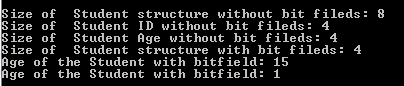# Bit Fields in C programming

## Bit Fields

Suppose we have a Student structure with his ID and age like below.

```typedef struct Student {
int StdId;
int Age
}STD;```

Here StdId is declared as integer and it usually unsigned value. Hence it will occupy 8 bytes of memory. Similarly Age is also an positive integer and hence occupies 8 bytes of memory. In practice, both Id and Age will not occupy 8 bytes of memory. It requires only less memory than what integer is supposed to occupy. Suppose student Id starts from 1 and it can take max of 100. Then it actually stored as 1100100 which is a 8 bits – 1 byte of memory. But we are creating a space for 8 bytes. This is a waste of memory. In order to efficiently manage the memory being used by the program, C allows us to limit the memory space occupied by the integer variables to bits. i.e.;

```typedef struct Student {
int StdId : 8;
int Age: 4;
}STD;```

Above code tells the compiler that even though StdId is declared as integer and can occupy 8bytes of space, limit the memory occupied by it to 8 bits or 1 byte. Similarly age is limited to 4 bits. Even though it looks lesser than its original memory size, it can store huge number of data value. That means 8 bits can store 28 values in it, which is more than enough for us now. Similarly, age can store 24 values in it.

For example, we can store age from 0 to 16 and student id from 0 to 256.

Since we have limited the number of values to lesser value, we cannot store any value greater than this. That means, if we try to store age as 17 or more, it will not allow us to store them. It will consider them as zero.

The general syntax for storing bit field variables is :

```struct {
type member_variable_name: size_in_bits;
}```

Where type is any datatype like int, char, float etc. member_variable_name is the member name of the structure and size_in_bits is the defined size of the member within the structure.

In above student structure size of the structure without bit field is size of (StdId) + size of (Age) = 8 bytes + 8 Bytes = 16 bytes. After using bit fields to its members, it is 8 bits + 4 bits = 12 bits = 1.5 bytes which is very much less. Hence we can save lot of memory.

```#include

void main(){
typedef struct Student_NoBit {
int StdId;
int Age;
}STD_NOBIT;

typedef struct Student {
int StdId : 8;
unsigned int Age : 4;
}STD;

STD_NOBIT std_nb;
STD std;

printf("\nSize of  Student structure without bitfield: %d\n", sizeof(std_nb));
printf("Size of  Student ID without bitfield: %d\n", sizeof(std_nb.StdId));
printf("Size of  Student Age without bitfield: %d\n", sizeof(std_nb.Age));
printf("Size of  Student structure with bitfield: %d\n", sizeof(std));

std.Age = 15; // this member can store max of 15 numbers from 0-15
printf("Age of the Student with bitfield: %d\n", std.Age);

std.Age = 17; // Since it is more than 15, it starts displaying the remainder values
printf("Age of the Student with Bitfield: %d\n", std.Age);
}```Please note that we cannot use sizeof operator to find the size of variable whose size is in bits. Please note above the difference between the structures with and without bitfields. Also see that how the member elements display the value based on their bits. Here Age can store values from 0 to 15 as its bit field size is 4. Hence it displays the values correctly till it has value 15. Above this number it shows the remainder values when tried to display.

Translate »